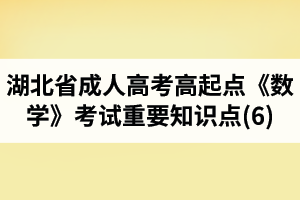# 湖北省成人高考高起点《数学》考试重要知识点(6)●知识点磁场

(★★★★★)已知函数f(x)=ax3+bx2+cx+d的图象如图，求b的范围。

●知识点磁场

(★★★★★)设函数f(x)的定义域为R，对任意实数x、y都有f(x+y)=f(x)+f(y)，当x>0时f(x)<0且f(3)=-4。

(1)求证：f(x)为奇函数;

(2)在区间[-9，9]上，求f(x)的最值。

●知识点磁场

(★★★★)已知α、β为锐角，且x(α+β- )>0，试证不等式f(x)= x<2对一切非零实数都成立。

●案例探究

[例1]设z1=m+(2-m2)i，z2=cosθ+(λ+sinθ)i，其中m，λ，θ∈R，已知z1=2z2，求λ的取值范围。

●知识点磁场

(★★★★★)已知 <β<α< ，cos(α-β)= ，sin(α+β)=- ，求sin2α的值_________.

### 湖北成人高考新手指南

 成考简介 报考条件 报考费用 考试时间 考试科目 招生简章 录取结果 阅卷工作 准考证打印

### 湖北成人高考网专题